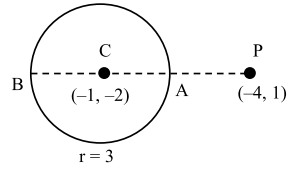# Solve the Following Questions`
Question:

Let $r_{1}$ and $r_{2}$ be the radii of the largest and smallest circles, respectively, which pass through the point $(-4,1)$ and having their centres on the circumference of the circle $x^{2}+y^{2}+2 x+4 y-4=0$.

If $\frac{r_{1}}{r}=a+b \sqrt{2}$, then $a+b$ is equal to :

1. 3

2. 11

3. 5

4. 7

Correct Option: , 3

Solution:Centre of smallest circle is A

Centre of largest circle is B

$r_{2}=|C P-C A|=3 \sqrt{2}-3$

$r_{1}=C P+C B=3 \sqrt{2}+3$

$\frac{\mathrm{r}_{1}}{\mathrm{r}_{2}}=\frac{3 \sqrt{2}+3}{3 \sqrt{2}-3}=\frac{(3 \sqrt{2}+3)^{2}}{9}=(\sqrt{2}+1)^{2}=3+2 \sqrt{2}$

$a=3, b=2$